Related Articles

# Rolling Motion

• Last Updated : 30 Jun, 2021

Consider extended bodies to be rigid bodies, and you can solve a lot of problems with them. A rigid body is one that has a precisely defined and unchanging shape. The distances between all pairs of particles in a body like this remain constant. Because real bodies flex under the impact of forces, this definition of a rigid body shows that no real body is genuinely rigid. However, in many cases, the deformations are insignificant. On the other hand, we can overlook the fact that bodies like wheels, tops, steel beams, molecules, and planets warp (twist out of shape), bend, or vibrate in a variety of settings and perceive them as solid.

The rolling motion is one of the most prevalent movements seen in everyday life. There is a rolling motion in all wheels used in transportation like cars, buses, trains, airplanes, bikes, buffalo carts and there are so many things that have wheels attached to them like trollies. For clarity let’s begin with the example of a disc, but the result applies to any rolling body rolling on a level surface. It is assumed that the disc isn’t slipping when it rolls. This implies that the bottom of the disc in touch with the flat surface is at rest on the surface at any given time.

### What is Rolling Motion?

The rolling motion contains two types of motion, or it is a combination of two types of motion translational and rotational motion. The translational motion of a body is the movement of the center of mass.

During a body’s rolling motion, the surfaces which come into contact get to deform slightly, and that deforms is temporary that is when the area of both bodies comes into touch with each other, results in temporary deformation of the bodied. This phenomenon has the impact effect called friction that is the component of the contact force parallel to the surface that resists the motion.

(1) Translatory motion

Translatory motion is a sort of motion in which all portions of the body move the same distance in the same amount of time. There are two forms of translation motion: rectilinear and curvilinear.

• A motion is said to be in rectilinear motion when a body in translatory motion moves in a straight line. For example, a car moving on the straight road, a train running on a straight track.
• The curvilinear motion describes the movement of a body in translatory motion moves along a curved path. For example, turning a car.

(2) Rotational motion

The motion of a body in a circular path around a fixed point in space, also, the motion of a body that does not deform or change shape in which all of its particles move in circles around an axis with a common angular velocity. For example the motion of the earth about its own axis, the motion of a wheel of vehicles, gears, motors, etc.

Expression for Rolling Motion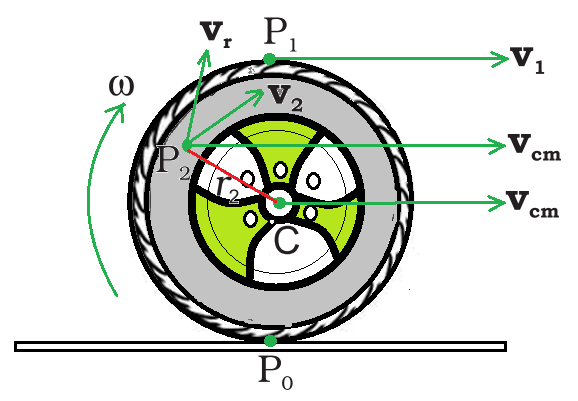The rolling motion (without slipping) of a wheel on a level surface.

Let the velocity of the wheel’s center of mass is vcm, which is its translational velocity. Because the rolling wheel’s center of mass is at its geometric center C, vcm is the velocity of C. It is parallel to the flat surface. The wheel rotates along its symmetrical axis, which passes through the center of C. As a result, the velocity of each point on the disc, such as P0 and P1, or P2, is made up of two parts that are the translational velocity vcm and the linear velocity vr due to rotation.

• The magnitude of vr is vr = ωr, where r is the distance between the point and the axis and ω is the angular velocity of the wheel rotation about the axis. With regard to C, the velocity vr is perpendicular to the radius vector of the given point w.r.t C. vr is perpendicular to CP2 in this case.
• It’s simple to demonstrate that v2 is perpendicular to the line P0P2. As a result, the instantaneous axis of rotation is defined as a line passing through P0 and parallel to ω.
• Because of rotation, the linear velocity, vr is exactly opposite the translational velocity, vcm, at point P0. Furthermore, the magnitude of vr, in this case, is Rω, where R is the wheel’s radius.
• The condition that P0 is instantaneously at rest requires vcm = Rω. Thus, for the wheel, the condition for rolling without slipping is vcm = Rω.
• This also means that the velocity of point P1 at the top of the wheel v1 is vcm + R or 2 vcm and is parallel to the level surface.

### Kinetic Energy of Rolling Motion

The kinetic energy of a rolling body can be divided into two types: translational and rotational kinetic energy.  The kinetic energy of a system of particles (K) can be divided into the kinetic energy of center-of-mass motion (translation) (MV2/2) and the kinetic energy of rotational motion about the system’s center of mass (K′). Therefore,The kinetic energy of the rolling body’s center of mass, i.e., the kinetic energy of translation, is m(vcm)2/2, where m is the body’s mass and vcm is the center of mass velocity.

The motion of the rolling body about its center of mass K′ is the kinetic energy of rotation of the body K′ = Iω2/2, where I is the moment of inertia about the suitable axis, which is the rolling body’s symmetry axis. Therefore, the kinetic energy of a rolling body is given bySubstitute I = mk2 where k is the corresponding radius of a rapidly moving body and ω=vcm/R where R is the radius of the circular moving body.### Types of Motion of a Rigid Body

Let’s look at some examples of rigid body motion. Let’s start with a rectangular block that slides down an inclined plane without moving sideways. The block is treated as if it were a rigid body. Its travel down the plane is such that all the body’s particles are moving in lockstep, i.e. they have the same velocity at all times. The rigid body is in pure translational motion in this scene.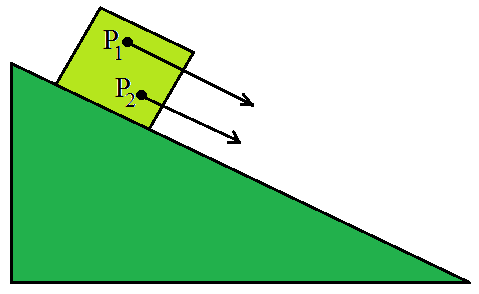Translational (sliding) motion of a block down an inclined plane. (Any point like P1 or P2 of the block moves with the same velocity at any instant of time.)

• Consider how a solid metallic or wooden cylinder might roll down the same slanted plane.  The cylinder, shifts from the top to the bottom of the inclined plane, giving the impression of translational motion. However, as seen in all of its particles are not traveling at the same speed at any given time. As a result, the body is not moving in a true translational motion. It moves in a combination of a translational and something else motion.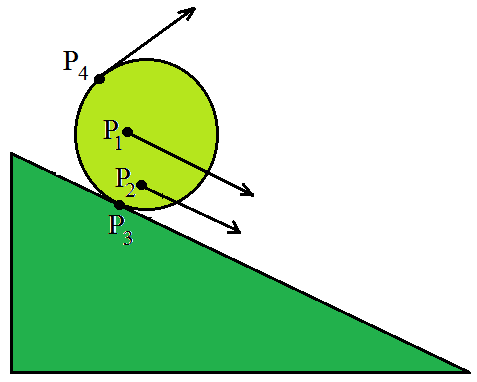The rolling motion of a cylinder. It is not pure rapture translational motion. Points P1, P2, P3, and P4 have different velocities (shown by arrows)at any instant of time. In fact, the velocity of the point of contact P3 is zero at any instant, if the cylinder rolls without slipping.

• Fixing a rigid body along a straight line is the most common approach to prevent it from having translational motion. Rotation is the only feasible motion for such a stiff body. The axis of rotation is the line or fixed axis around which the body rotates. For example, a ceiling fan, a potter’s wheel, a giant wheel in a fair, and so on. When a rigid body rotates around a fixed axis, each of its particles moves in a circle that exists in a plane perpendicular to the axis and has its center on the axis. However, in rare cases of rotation, the axis may not be fixed. A top spinning in place is a good example of this type of rotation. Through its point of contact with the ground, the axis of such spinning top swings around the vertical, sweeping out a cone.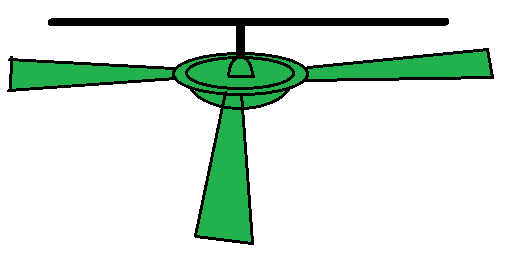Rotation about a fixed axis a ceiling fan.

• If a rigid body is not pivoted or attached in any way, its motion is either pure translation or a combination of translation and rotation. The motion of a rigid body that is pivoted or fixed in some way is called rotation. The rotation could be fixed, such as with a ceiling fan, or moving, such as with an oscillating table fan.A spinning top

### Sample Problems

Problem 1: Three bodies, a ring, a solid cylinder, and a solid sphere roll down the same inclined plane without slipping. They start from rest. The radii of the bodies are identical. Which of the bodies reaches the ground with maximum velocity?

Solution:

According to the conservation of energy of the rolling body, i.e. there is no loss of energy due to friction. . The potential energy lost by the body in rolling down the inclined plane is P.E= mgh, therefore, be equal to kinetic energy gained. The bodies start from rest the kinetic energy gained is equal to the final kinetic energy of the bodies.

The expression for the kinetic energy iswhere v is the final velocity of the Centre of mass of the body.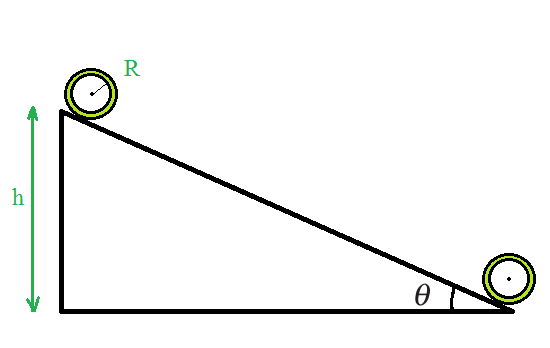Equate K and mgh,Here, velocity is independent of the mass of the rolling body,

For a ring, k2= R2, therefore the expression can be written asFor a solid cylinder k2= R2/2 , therefore,Now for a solid sphere k2= 2R2/5 , therefore,The results show that the sphere has the greatest and the ring has the least velocity of the centre of mass at the bottom of the inclined plane.

Problem 2: Find the moment of inertia of a sphere about a tangent to the sphere, given the moment of inertia of the sphere about any of its diameters to be 2 MR2/5, where M is the mass of the sphere and R is the radius of the sphere. (b) Given the moment of inertia of a disc of mass M and radius R about any of its diameters to 1 be 1/4 MR2, find the moment of inertia about an axis normal to the disc passing through a point on its edge.

Solution:

(a) Moment of inertia of sphere about any diameter = 2/5 MR2

Applying theorem of parallel axes,

Moment of inertia of sphere about a tangent to the sphere = 2/5 MR2 +M(R)2

=7/5 MR2

(b) Given,

The expression for the moment of inertia of the disc about any of its diameters can be written as

L= 1/4 MR2

(i) Using theorem of perpendicular axes, the moment of inertia of the disc about an axis passing through its centre and normal to the disc can be written as

I= 2 x 1/4 MR2

I = 1/2 MR2.

(ii) Using theorem axes, moment of inertia of the disc passing through a point on its edge and normal to the dies can be written as

I = 1/2 MR2+ MR2

I = 3/2 MR2.

Problem 3: Equal magnitude of torque is applied to a hollow cylinder and a solid sphere, both having the same mass and radius. The cylinder is free to rotate about its standard axis of symmetry, and the sphere is free to rotate about an axis passing through its center. Which of the two will acquire a greater angular speed after a given time?

Solution:

Let the radius of the hollow cylinder, and the solid sphere be R and the mass M .

The moments of inertia of the hollow cylinder about the respective axes is I1 = MR2

And the moments of inertia of the solid sphere about the respective axes I2 = (2/5) MR2

Let the magnitude of the torque applied to the cylinder and the sphere is τ , Which produce angular accelerations α1 and α2

Then, the magnitude of the torque can be expressed as

τ = I1 α1 = I2 α2

The sphere is larger angular acceleration. Therefore, the sphere will acquire larger angular speed after a given time.

Problem 5: A ring of mass m and radius R has three particles attached to the ring as shown in the figure. The center of the ring has a speed v0. If the kinetic energy of the system is xmv0 2. Find x (Slipping is absent)

Solution:

The expression for the rolling motion wen slip is absent is,Now the speed of the 2m particle placed at left side isThe speed of the m particle placed at right side isThe speed of the m particle placed at top isThe kinetic energy placed at left , right and top isKinetic energy of the ring is

K.E = rotational kinetic energy + translational kinetic energy.Substitute  I = mR2 and ω = v0/R in the above equation,The total kinetic energy isTherefore, the value of x is 6.

Attention reader! Don’t stop learning now. Join the First-Step-to-DSA Course for Class 9 to 12 students , specifically designed to introduce data structures and algorithms to the class 9 to 12 students

My Personal Notes arrow_drop_up